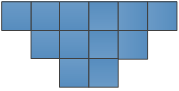### Sample Problem

The figure below is made up of squares with side length 2 cm. What is the area of the figure?

cm2#### Solution

Area of 1 square = 2 cm × 2 cm

= 4 cm2

Number of squares = 12

∴ Area of figure = 12 × 4 cm2

= 48 cm2

The area of the figure is 48 cm2.